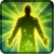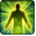# Scatter SporesConditions

## Related effects, buffs and debuffs

Please click on an effect below to view its details.

• [0s] [not displayed]Effect #1

 Slot: Buff Duration: 0s Tick rate: does not tick # occurrences: 0
• On Apply
• [target override]

Perform the following actions:

• Add effect #2 to TARGET from TARGET
• [hidden] [15s]Effect #2

 Slot: Buff Duration: 15s Tick rate: does not tick # occurrences: 0 Conditions: Can only by called by other effects
• On Apply

Perform the following actions:

• Unknown (183)
- Unknown (303) = (bool) true
- Unknown (306) = (bool) false
- Unknown (430) = (bool) false
- Unknown (310) = (string) aoePositionOptionType_Entity
- Unknown (311) = (string) aoeTarget_Ground
- Unknown (313) = (string) aoeScalarType_LiteralValue
- Unknown (317) = (string) aoeScalarType_LiteralValue
- Unknown (321) = (string) aoeScalarType_LiteralValue
- Unknown (325) = (string) aoeOrientationOptionType_WorldCoordinates
- Unknown (344) = (string) aoeSphere
- Unknown (349) = (string) aoeTargetCondition_IsPlayer
- Unknown (353) = (string) aoeScalarType_LiteralValue
- Unknown (354) = (string) aoeScalarType_LiteralValue
- Unknown (355) = (string) aoeScalarType_LiteralValue
- Unknown (367) = (string) aoeScalarType_LiteralValue
- Unknown (384) = (string)
- Unknown (386) = (string) aoeAbilityCaster_Caster
- Unknown (387) = (string) aoeAbilityTarget_Target
- Unknown (388) = (string)
- Unknown (389) = (string)
- Unknown (431) = (string) aoeRotation_None
- Effect Number = (int) 0
- Ability Spec = (int) 16140956060617984365
- Unknown (307) = (int) 1
- Unknown (308) = (int) 1
- Tick Rate = (float) 1
- Unknown (304) = (float) 45
- Unknown (305) = (float) 45
- Unknown (314) = (float) 0
- Unknown (318) = (float) 0
- Unknown (322) = (float) 0
- Unknown (362) = (float) 0
- Unknown (363) = (float) 0
- Unknown (364) = (float) 0
- Unknown (368) = (float) 0.8
- Unknown (390) = (float) 0
- Unknown (398) = (float) 0.1
• On Apply

Perform the following actions:

• Play appearance epp . operation . dxun . boss . the_pack_leader . mechanics . phosphilapse_corona . spore_radius, dependent on calling effect